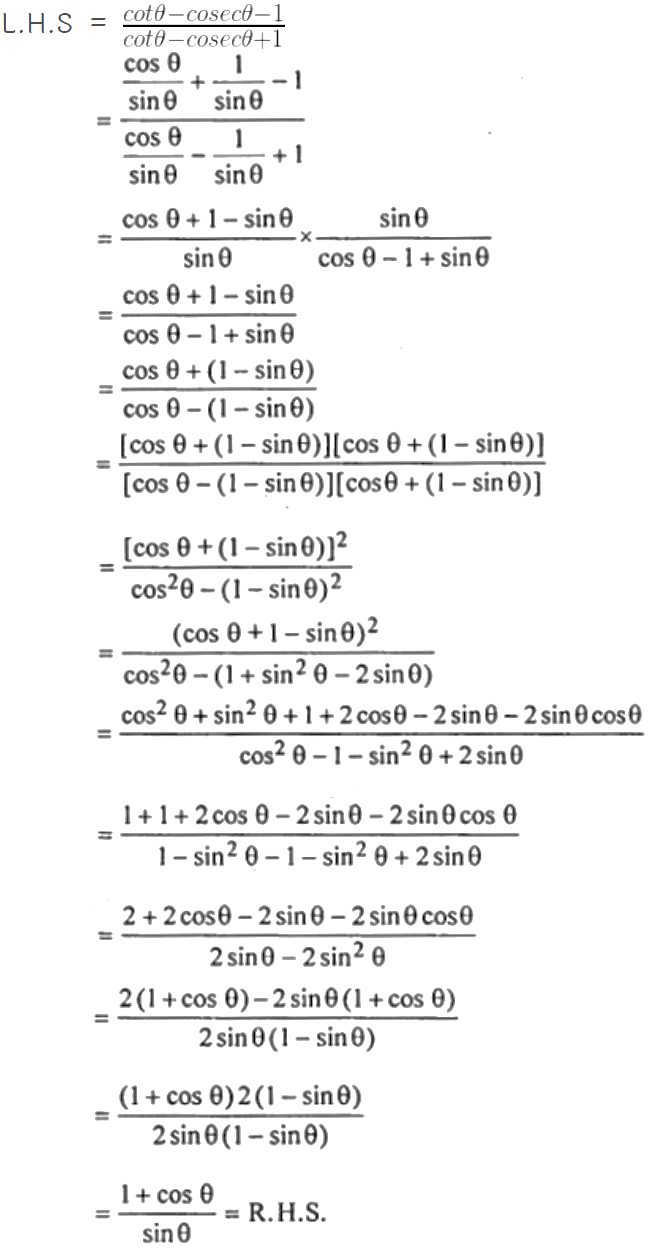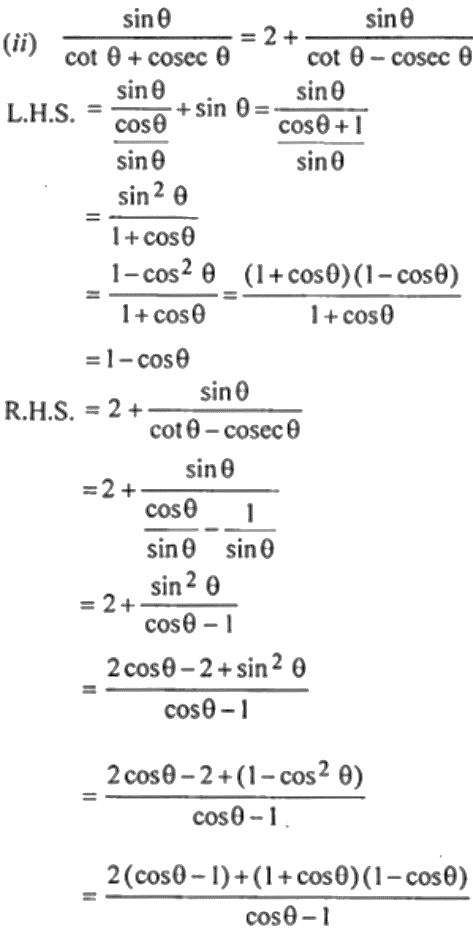Guru

# (i) (Cot theta +cosec theta-1) /(cot theta – cosec theta+1) =(1+cos theta) /sin theta (ii) Sin theta/cot theta+cosec theta = 2+ sin theta/cot theta-cosec theta

• 1

This is an important question from the
Book- ML Aggarwal
Board- ICSE
Publication- Avichal
Chapter- Trigonometric Identities
Chapter number-18

We have to prove that

(i) (Cot theta +cosec theta-1) /(cot theta – cosec theta+1) =(1+cos theta) /sin theta (ii) Sin theta/cot theta+cosec theta = 2+ sin theta/cot theta-cosec theta

Trigonometric Identities, class 10th, chapter 18th, question no27 , ICSE board, ML aggarwal..

Share

1. Solution:

(i)= (cos θ – 1) (2 – 1 – cos θ)/ (cos θ – 1)

= 1 – cos θ

Hence, L.H.S = R.H.S.

• 0